Home > INT3 > Chapter 7 > Lesson 7.2.5 > Problem7-117

7-117.

Use the functions f(x) = 3x2 – 5 and g(x) = x – 3 to complete the parts below. Homework Help ✎

1. What is f(–5)?

2. Write an expression for 7g(x).

3. What is f(4)?

4. Write an expression for g(3x).

5. Write an expression for f(x + 4).

6. Write an expression for g(x) – f(x).

7. Describe the domain of f(x).

8. Describe the domain of g(x).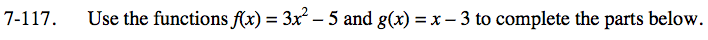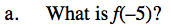Substitute −5 for x.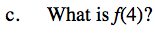43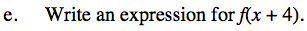Substitute x + 4 for x in the expression.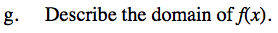all real numbers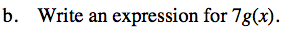Multiply and distribute 7 by the g(x) expression.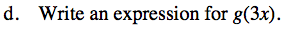3x − 3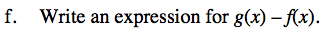Remember to distribute the negative sign to all terms in f(x).

−3x2 + x + 2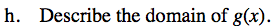See part (g).